# Electronics and Communication Engineering - Electromagnetic Field Theory

46.
A broadside array is shown in figure in which the elements are placed at equal distances. The direction of maximum radiation is represented by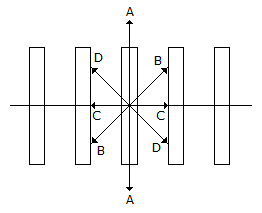AA
BB
CC
DD
Explanation:
No answer description is available. Let's discuss.

47.
Which one of the following is correct expression. Where t is torque, B magnetic field, M is the moment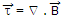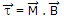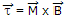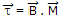Explanation:
No answer description is available. Let's discuss.

48.
There is no radiation at right angles to the plane of the array, is end fire array.
True
False
Explanation:
No answer description is available. Let's discuss.

49.
Which of the following constitute the loss resistance of an antenna?
Dielectric loss
Leakage loss in insulation
Loss in earth connections
All of the above